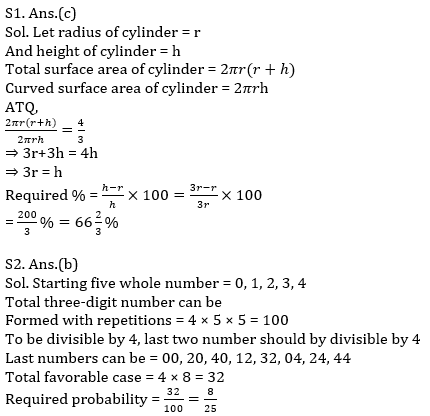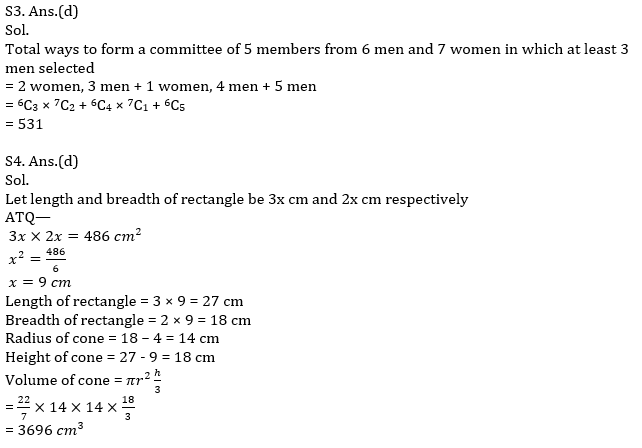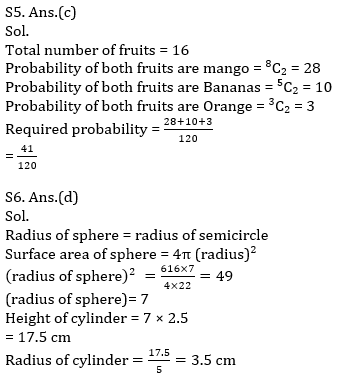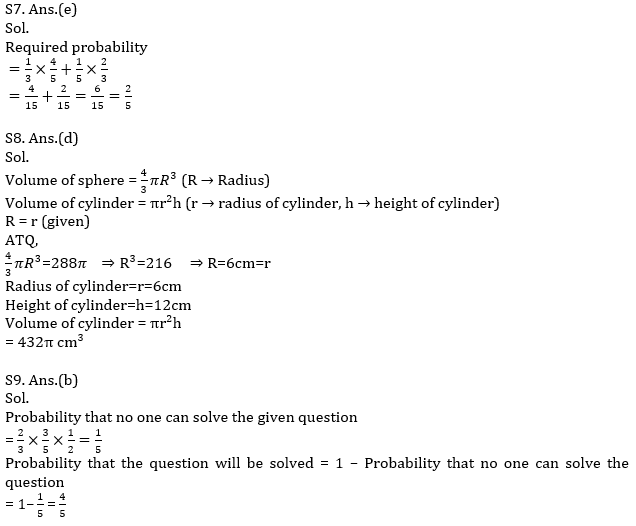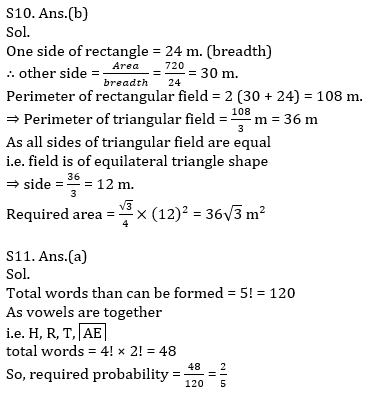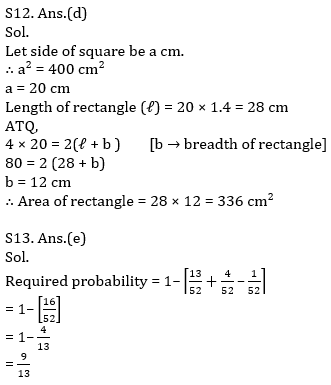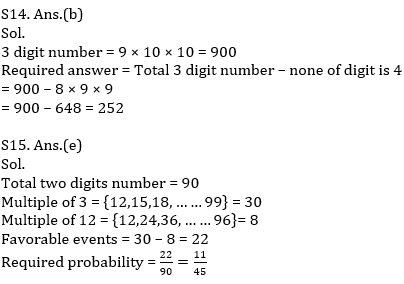Latest Banking jobs   »   Quantitative Aptitude Quiz For IBPS RRB...

# Quantitative Aptitude Quiz For IBPS RRB PO, Clerk Prelims 2021- 28th June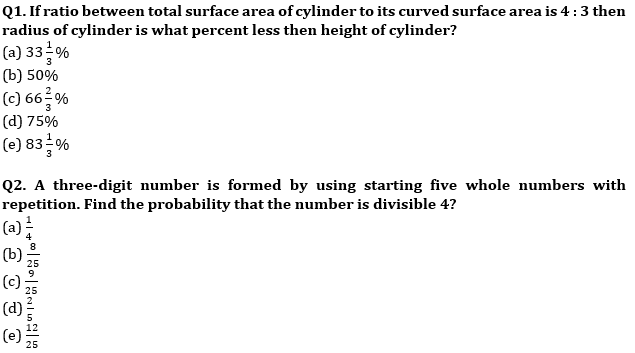Q3. In how many ways a committee of 5 member can be formed from 6 men 7 women in which at least 3 men should come:
(a) 431
(b) 520
(c) 720
(d) 531
(e) 640

Q4. Ratio between length and breadth of a rectangle is 3 : 2 and the area of rectangle is 486 cm2. If breadth of rectangle is 4 cm more than radius of a cone and length of rectangle is 9 cm more than height of the cone, then find the volume of cone?
(a) 3996 cm³
(b) 4096 cm³
(c) 3896 cm³
(d) 3696 cm³
(e) 3998 cm³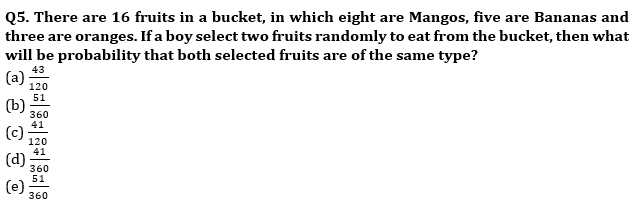Q6. The radius of a semicircle is equal to the radius of a sphere whose surface area is 616 cm² and height of a cylinder is 150% more than radius of semicircle and ratio of height to radius of cylinder is 5 : 1. Then find radius of cylinder? (in cm³)
(a) 14
(b) 7.5
(c) 3
(d) 3.5
(e) 5.5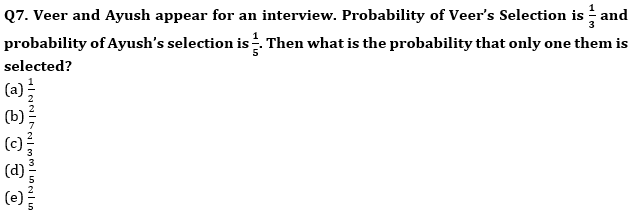Q8. The radius of a cylinder & a sphere is same and ratio of height and radius of cylinder is 2 : 1.If the volume of sphere is 288 π cm³ then find the volume of cylinder? (in cm³)
(a) 438 π
(b) 426 π
(c) 420 π
(d) 432 π
(e) 444 π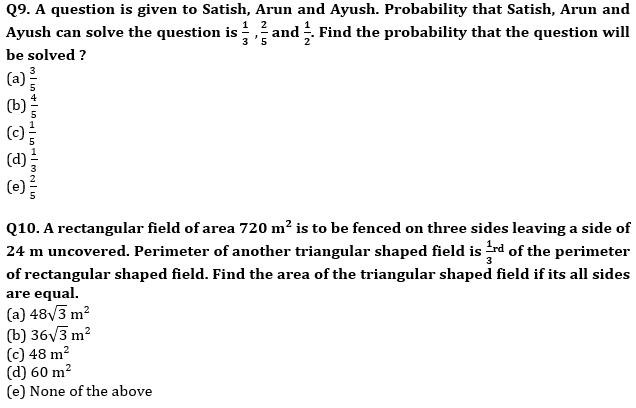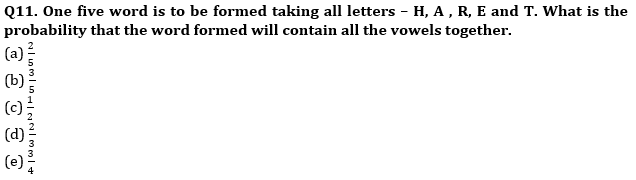Q12. Perimeter of rectangle is equal to the perimeter of square whose area is 400 cm² and length of rectangle is 40% more than the side of a square then find the area of rectangle?
(a) 248 cm²
(b) 420 cm²
(c) 356 cm²
(d) 336 cm²
(e) 348 cm²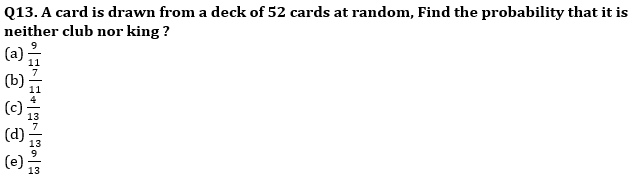Q14. Find the number of 3-digit numbers such that they have atleast one digit which is 4 (with repetition)
(a) 332
(b) 252
(c) 298
(d) 216
(e) 328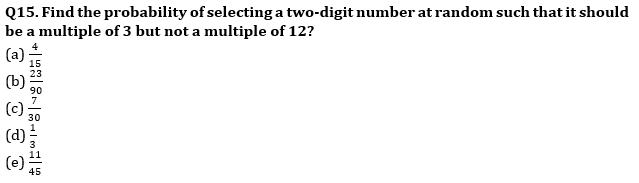Practice More Questions of Quantitative Aptitude for Competitive Exams:

###### Study Plan for IBPS RRB PO/Clerk Prelims 2021

Solutions